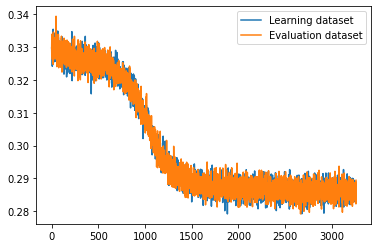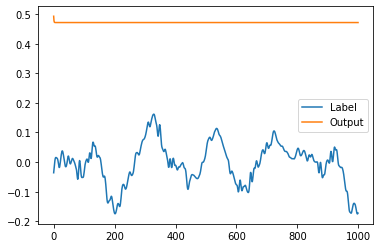# Lstm time series prediction flat line output, loss stagnation

I am trying to train an lstm model for music generation, and now i am at a stage of “Get somewhat sane results out of that pile of algebra”

At first i tried a model of 1 LSTM -> 1 linear layer, and loss fell to ~0.02 and then remained stable, but then, when i tried to do 2LSTM -> 2 linear layers, loss didnt fall lower than ~0.2, but i expected it to be even lower, because of increased model complexity

2LSTM -> 2Linear graph (sampled every batch, 1988 batches/epoch):Classes:

``````class LSTMModule(nn.Module):
"""
Wrapper for nn.LSTM for easier understanding
"""
def __init__(self, in_features = 1, out_features = 1, num_layers = 1, activation = None, device = 'cuda'):
super().__init__()
self.lstmlayer = nn.LSTM(in_features,out_features,num_layers).to(device)
self.activation = activation
self.device = device

def forward(self, input_val, hidden = None):
input_val = input_val.to(self.device)
if hidden is not None:
hidden = hidden.device(self.device)
output,hidden = self.lstmlayer(input_val, hidden)
#if self.activation is not None:
#output = self.activation(output) #turns out, tanh is built into LSTM, nice
return output, hidden

class LinearModule(nn.Module):
"""
Module for sequence processing with nn.Linear layers
"""
def __init__(self,in_features = 1, out_features = 1, batch_first = False, num_layers = 1, activation = None, device = 'cuda'):
super().__init__()
if num_layers == 0:
raise Exception("Linear module cant have 0 layers")
self.layers = [nn.Linear(in_features,out_features).to(device) for i in range(0,num_layers)]
self.activation = activation
self.device = device
self.batch_first = batch_first

def forward(self, input_val):
if not self.batch_first: #reshape into (batches,seq_length,features), if not already
output = input_val.reshape(input_val.size(1),input_val.size(0),1)
else:
output = input_val

output = output.to(self.device)
for i in range(0,len(self.layers)): #iterate over all layers in module, 2 in my case
output = self.layers[i](output)
if self.activation is not None:
output = self.activation(output)
return output

class network(nn.Module):
def __init__(self, input_size=1, hidden_layer_size=1, output_size=1, seq_length_ = 1, batch_size_ = 128):
super().__init__()
#self.hidden_layer_size = hidden_layer_size
#self.batch_size = batch_size_
#self.seq_length = seq_length_

self.memory_module = LSTMModule(device = DEVICE, num_layers = 2)
self.linear_module = LinearModule(activation = nn.Tanh(),device = DEVICE, num_layers = 2)

def forward(self, input_seq):
lstm_output, _ = self.memory_module(input_seq)
output = self.linear_module(lstm_output)
return output.reshape(output.shape,output.shape,1) #reshape back to seq,batch,features
``````

Is there something wrong with how my model is structured (module in module in model, iterating over linear layers in for loop), or is it just a local minima?

UPD:
Looked at models output, it is just a flat line:I am starting to feel like i am doing something wrong

Hey, Topsoil!

Have you managed to figure out a solution to your problem?
I’m having the exact same issue for the past few weeks…

Sorry for not replying, i left my ML hobby for a few months, if it is still useful, the problem was probably in a moduled structure, because after i rewrote it from scratch but using just layers, it started working, kinda, still didnt output any useful results, but output was at least somewhat structured, sorry once again for not replying

1 Like

All good!